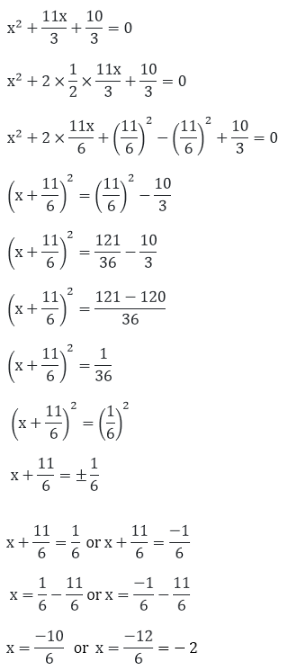Newbie

# Find the roots of the following quadratic equations (if they exist) by the method of completing the square. 3. 3×2 + 11x + 10 = 0

• 0

Explain, how did we split the number in the equation.

How to prove?

Class 10th, Statistics, Rd sharma, Mathematics.

Share

1.3. 3x2 + 11x + 10 = 0

Solution:

Given equation,⇒ x = -5/3 = 3 or x = -2

Thus, the roots of the given quadratic equation are x = -5/3 and x = -2.

• 0